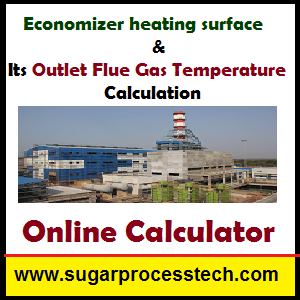# Economizer heating surface and Its Outlet Flue Gas Temperature Calculation

## How to find the economizer heating surface  in thermal power plants

The combustion gases leave the boiler at a temperature which is still relatively high, and generally above the saturation temperature. This sensible heat content of the gases would be lost in the stack. Hence the idea of utilizing their sensible heat content to raise the temperature of the feed water, and so decrease the quantity of heat to be supplied to the steam in the boiler.

Principle of the economizer:  It is a heat exchanger placed in the path of the flue gases leaving the boiler, and through which the feed water is circulated between the feed pump and the boiler. It generally takes the form of tubes, in most cases with fins, through which the water circulates in series. They are arranged in groups, the water passing from one tube to the following by means of a 180oC bend.

### ECONOMIZER HEATING SURFACE CALCULATION

#### Data to be required for calculation:

Steam production  ( Qs ) in Kg/hr

Flue gas inlet temperature ( To ) in oC

Water  Quantity  ( Qw ) in Kg/hr ( It is equal to steam production)

Water inlet temperature (to ) ) in oC

Water outlet temperature ( t ) ) in oC

Steam & fuel ratio  (R )

Heat transfer coefficient In economizer  ( K )  (Note : As per E. Hugot this value having  20 to 30 Kcal/m2/hr/oC)

Solid unburden coefficient   ( α )

Moisture of bagasse ( W ) in %

Excess air  (m) in %

#### Calculation Part :

Weight of air required per kg of Bagasse  (Pa)=  5.76 (1-W) m

Weight of the gaseous products of combustion per kg of bagasse ( A ) = 5.76(1-W)m + 1

Fuel consumption ( F ) = Qs / R

Total air requirement   (P) =   Px F

Flue gas Quantity (Pg) = F X A

Specific heat of water ( Cw) =  1  Kcal/Kg/oC

Specific heat of flue gas  ( CF) = 0.27+0.00006T in Kcal/Kg/oC

Ratio ( r ) ==Ratio r = This is the ratio (t —to)/ (To — T ) between the rise in temperature of the water and the drop in temperature of the gases. It varies between 0.6 and 0.8; that is, the water temperature increases by 0.7° when the gases drop by 1°.

Flue gas outlet temperature ( T  ) in oC = To –Heating Surface   ( S ) in m2While find the Heat transfer coefficient of our existing system

Heat transfer coefficient K  in Kcal/m2/hr/oC =x### Online Calculation for find Economizer heating surface and Its Outlet Flue Gas Temperature

(Note : This Calculator is very helpful to cross check of our exiting system )

Related Articles:

Concepts of milling tandem like Preparatory Index, Primary and secondary extractions, roller grooving, hydraulic load , mill settings, imbibition etc in sugar industry.

Need of Boiler Feed water Treatment | Boiler water treatment process | Boiler feed water specification.

Methods for Industrial Effluent Treatment Plant (ETP).

Direct Contact Heater (DCH) Important features, formulas and considerations in design calculation with Online calculator.

Hi friends Thanks for reading. I Hope you liked it. Give feed back, comments and please share it

## 6 thoughts on “Economizer heating surface and Its Outlet Flue Gas Temperature Calculation”#### yohannis hussen

(May 27, 2018 - 8:35 pm)

i wanna know about design calculations of economizer#### NomAn Ashraf

(January 26, 2021 - 7:54 am)

How we Check thek Value Of Ln?#### M Tayyab

(June 7, 2018 - 6:36 am)

We want to calculate heat transfer from economizer and air heater and feed water economizer out let temp and stack temp and heat recover by economizer and air heater.#### M Tayyab

(August 30, 2021 - 6:24 am)

Plz send me economizer calculation